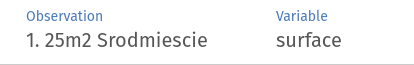## Setup

``````library(arenar)

apartments <- DALEX::apartments
``````

## Prepare models

Let's compare three models: GLM and GBMs with 100 and 500 trees. For each we create explainer from DALEX package.

``````library(gbm)
library(DALEX)
library(dplyr)

model_gbm100 <- gbm(m2.price ~ ., data = apartments, n.trees = 100)
expl_gbm100 <- explain(
model_gbm100,
data = apartments,
y = apartments\$m2.price,
label = "gbm [100 trees]"
)

model_gbm500 <- gbm(m2.price ~ ., data = apartments, n.trees = 500)
expl_gbm500 <- explain(
model_gbm500,
data = apartments,
y = apartments\$m2.price,
label = "gbm [500 trees]"
)

model_glm <- glm(m2.price ~ ., data = apartments)
expl_glm <- explain(model_glm, data = apartments, y = apartments\$m2.price)
``````

## Run arena

It is not necessary to use dplyr's pipes, but it's designed to use pipes.

``````arena <- create_arena(live = TRUE) %>%
# Pushing explainers for each models
push_model(expl_gbm100) %>%
push_model(expl_gbm500) %>%
push_model(expl_glm) %>%
# Push dataframe of observations
push_observations(apartments) %>%
# Run server of default port and ip
run_server()
``````

## Custom observation names

Oservations' names are taken from rownames. For example let's put district and surface as observation name.

``````apartments2 <- apartments
rownames(apartments2) <- paste0(
1:nrow(apartments2),
". ",
apartments2\$surface,
"m2 ",
apartments2\$district
)

create_arena(live = TRUE) %>%
push_model(expl_glm) %>%
push_observations(apartments2) %>%
run_server()
``````## Appending data

Sometimes you have already opened Arena session with static data, different live server or other data source. You can add new data without closing that window. Just use argument `append_data`.

``````create_arena(live = TRUE) %>%
push_model(expl_glm) %>%
run_server(append_data = TRUE)
``````